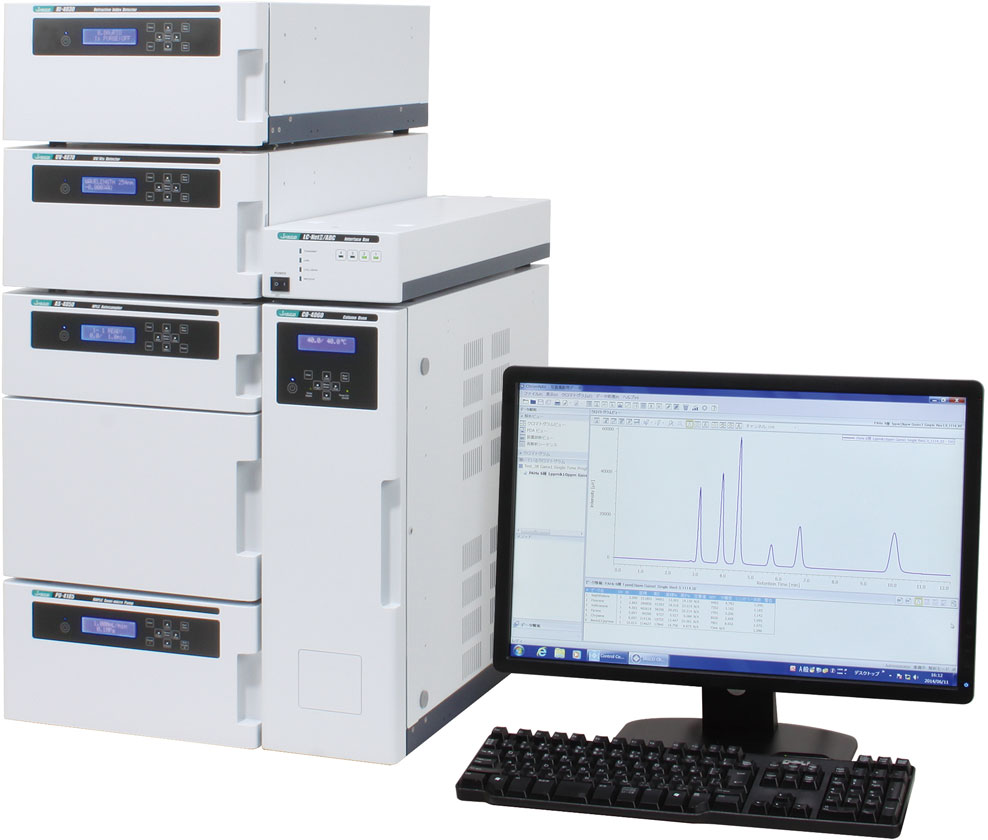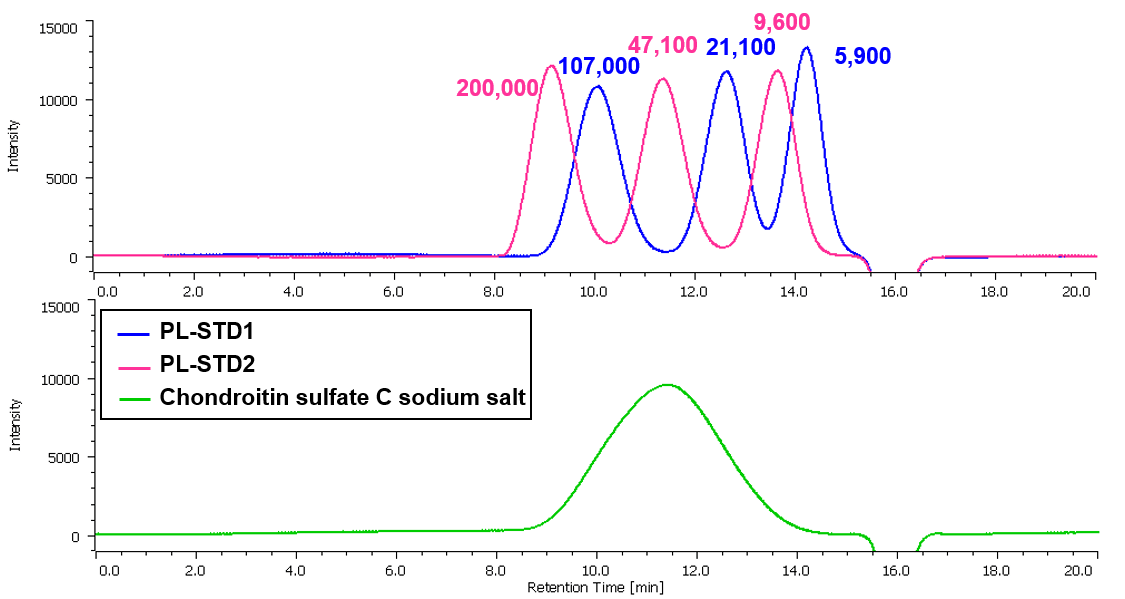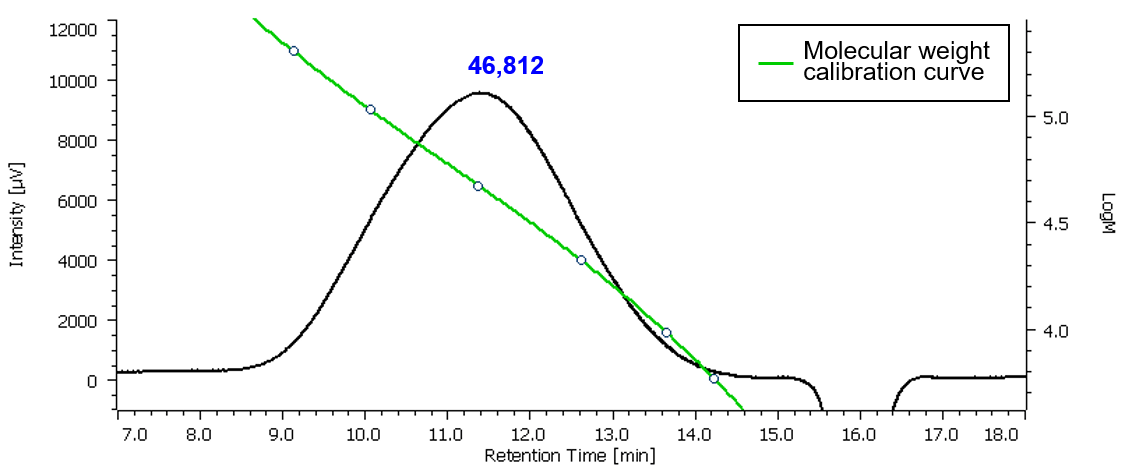# Analysis of Chondroitin Sulfate Sodium Salt by SEC

July 7, 2021

## Introduction

There are five kinds of chondroitin sulfate (MW 20,000 ~ 50,000) which is one of mucopolysaccharide, such as A~E, contained in connective tissues of animal’s cartilagines and skin, and they are used for drug medicines and healthy food. By using a column with exclusion limit of 300,000 molecular weight (Pullulan) for SEC (Size Exclusion Chromatography), chondroitin sulfate sodium salt was analyzed with an RI detector. The calibration curve was created with Pullulan as standard mixture by ChromNAV GPC Calculation Program (Optional) and the result calculated based on Pullulan converted molecular weight is shown below.LC-4000 GPC system

## Experimental

Chromatographic conditions
Column:  Shodex Asahipak GF-510 HQ (7.5 mmI.D. x 300   mmL, 5 µm)
Eluent:  0.04M Sodium dihydrogen phosphate anhydrous–   0.06M Disodium hydrogen phosphate anhydrous
Flow rate:   0.6 mL/min
Column temp.:  30ºC
Injection volume:  50 µL
Calibration standards for SEC:  Shodex STANDARD P-82 (Pullulan) 0.05% each in eluent

 PL-STD1 PL-STD2 Grade Mp Grade Mp P-100 107,000 P-200 200,000 P-20 21,100 P-50 47,100 P-5 5,900 P-10 9,600

Sample:  0.1% Chondroitin sulfate C sodium salt in eluent

Structure## Keywords

Chondroitin sulfate, SEC, RI detector, GPC Calculation Program

## Results

Figure 1 shows the chromatograms of Pullulan (PL), standard mixture for molecular weight calibration and chondroitin sulfate sodium salt.Figure 1. Chromatograms of Pullulan (PL), standard mixture for molecular  weight calibration and chondroitin sulfate sodium salt
(Each value on the chromatograms is Mp (Peak top molecular weight).)

Figure 2 shows molecular weight calibration curve created with Pullulan as a standard mixture. Figure 3 shows a chromatogram of chondroitin sulfate sodium salt and molecular weight calibration curve.  Figure 4 and Table 1 shows molecular weight distribution calculation curve and the result of molecular weight calculated with Pullulan respectively.Figure 2.  Molecular weight calibration curve created with PL standard mixtureFigure 3. Chromatogram of chondroitin sulfate sodium salt and molecular weight calibration curve
(The figure in blue represents Mp calculated with Pullulan)Figure 4. Molecular weight distribution curve of chondroitin sulfate sodium salt

Table 1. Pullulan converted molecular weight of chondroitin sulfate sodium salt

 Mp Mn Mw Mz Mv Mw/Mn Mz/Mw 46812 36668 57914 85947 57914 1.58 1.48

## Hint

Molecular weight calibration curve
This is to show the relationship between retention volume (elution time) and molecular weight, which is created to estimate the molecular weight of the sample from the retention volume (elution time) of the sample eluted from the column.

Molecular weight distribution curve
Integral molecular weight distribution curve is to show the relationship between molecular weight (logarithmic value) and a percentage against the total to indicate how much percentage of some molecular weight occupies against the total.

Differential molecular weight distribution curve is a curve where the molecular weight (logarithmic value) is plotted on the abscissa axis and the value of weight fraction differentiated by logarithmic value of molecular weight (dw/d(logM)),  on the ordinate axis. When normalized, such curve makes it possible to compare a chromatogram and a molecular weight distribution under the different columns and measurement conditions.

Molecular weight averages and polydispersity
The molecular weight averages of polymer materials obtained by size exclusion chromatography (SEC) includes the number-average (Mn), weight average (Mw), z-average (Mz), and viscosity-average (Mv) molecular weights.  These averages are defined by the following expressions.  The distribution of these molecular weight averages bears in general a relationship such as MnMvMwMz.  In case of Mn = Mv = Mw = Mz, there is no molecular weight distribution (monodispersed).* Ni represents the number of molecules for i component of molecular weight Mi  and a represents an index of Mark-Houwink-Sakurada equation.